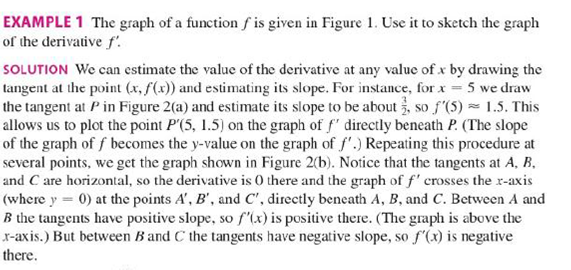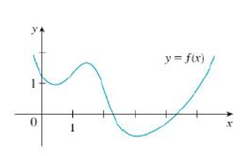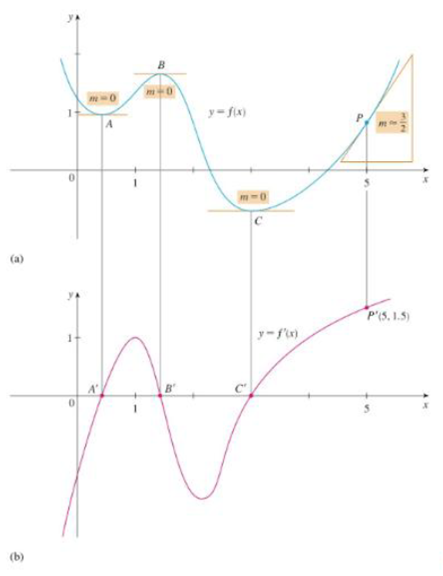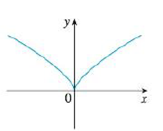Chapter 2.8, Problem 8E### Single Variable Calculus: Early Tr...

8th Edition
James Stewart
ISBN: 9781305270343

#### Solutions

Chapter
Section### Single Variable Calculus: Early Tr...

8th Edition
James Stewart
ISBN: 9781305270343
Textbook Problem

# Trace or copy the graph of the given function .f. (Assume that the axes have equal scales.) Then use the method of Example 1 to sketch the graph of f' below it.Example 1FIGURE 1FIGURE 2To determine

To sketch: The graph of f below the graph of f.

Explanation

From the point A to the left, the slope of the graph f is strictly negative which implies that the derivative graph f must have a functional value in negative.

From the given graph, it is observed that the graph has high sharpness at origin. So, there is no unique tangent line at origin. Thus, the given graph is not differentiable at (0,0). Therefore, the graph of f has a discontinuity at x=0

### Still sussing out bartleby?

Check out a sample textbook solution.

See a sample solution

#### The Solution to Your Study Problems

Bartleby provides explanations to thousands of textbook problems written by our experts, many with advanced degrees!

Get Started

#### Find more solutions based on key concepts